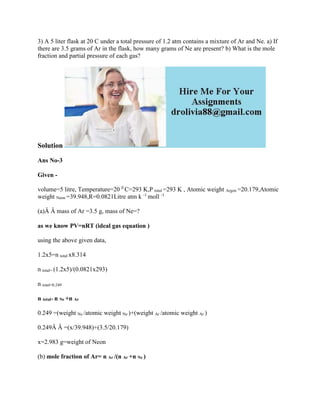Successfully reported this slideshow.

# 3) A 5 liter flask at 20 C under a total pressure of 1-2 atm contains.docx×

# 3) A 5 liter flask at 20 C under a total pressure of 1-2 atm contains.docx

3) A 5 liter flask at 20 C under a total pressure of 1.2 atm contains a mixture of Ar and Ne. a) If there are 3.5 grams of Ar in the flask, how many grams of Ne are present? b) What is the mole fraction and partial pressure of each gas?
Solution
Ans No-3
Given -
volume=5 litre, Temperature=20 0 C=293 K,P total =293 K , Atomic weight Argon =20.179,Atomic weight Neon =39.948,R=0.0821Litre atm k -1 moll -1
(a)Â Â mass of Ar =3.5 g, mass of Ne=?
as we know PV=nRT (ideal gas equation )
using the above given data,
1.2x5=n total x8.314
n total= (1.2x5)/(0.0821x293)
n total=0.249
n total= n Ne +n Ar
0.249 =(weight Ne /atomic weight Ne )+(weight Ar /atomic weight Ar )
0.249Â Â =(x/39.948)+(3.5/20.179)
x=2.983 g=weight of Neon
(b) mole fraction of Ar= n Ar /(n Ar +n Ne )
mole fraction of Ar=(weight Ar /atomic weight Ar )/((weight Ar /atomic weight Ar )+((weight Ne /atomic weight Ne )
mole fraction of Ar=(3.5/20.179)/((3.5/20.179)+(2.983/39.948))
mole fraction of Ar=0.248
mole fraction of Ne=(weight Ne /atomic weight Ne )/((weight Ar /atomic weight Ar )+((weight Ne /atomic weight Ne )
mole fraction of Ne=(2.983/39.948)/((3.5/20.179)+(2.983/39.948))
mole fraction of Ne=0.720
P partial= P total xmole fraction of particular component
P partial of Ar =1.2 xmole fraction of Ar
P partial of Ar =0.297 atm
P partial of Ne =1.2 xmole fraction of Ne
P partial of Ne =0.902 atm
.

3) A 5 liter flask at 20 C under a total pressure of 1.2 atm contains a mixture of Ar and Ne. a) If there are 3.5 grams of Ar in the flask, how many grams of Ne are present? b) What is the mole fraction and partial pressure of each gas?
Solution
Ans No-3
Given -
volume=5 litre, Temperature=20 0 C=293 K,P total =293 K , Atomic weight Argon =20.179,Atomic weight Neon =39.948,R=0.0821Litre atm k -1 moll -1
(a)Â Â mass of Ar =3.5 g, mass of Ne=?
as we know PV=nRT (ideal gas equation )
using the above given data,
1.2x5=n total x8.314
n total= (1.2x5)/(0.0821x293)
n total=0.249
n total= n Ne +n Ar
0.249 =(weight Ne /atomic weight Ne )+(weight Ar /atomic weight Ar )
0.249Â Â =(x/39.948)+(3.5/20.179)
x=2.983 g=weight of Neon
(b) mole fraction of Ar= n Ar /(n Ar +n Ne )
mole fraction of Ar=(weight Ar /atomic weight Ar )/((weight Ar /atomic weight Ar )+((weight Ne /atomic weight Ne )
mole fraction of Ar=(3.5/20.179)/((3.5/20.179)+(2.983/39.948))
mole fraction of Ar=0.248
mole fraction of Ne=(weight Ne /atomic weight Ne )/((weight Ar /atomic weight Ar )+((weight Ne /atomic weight Ne )
mole fraction of Ne=(2.983/39.948)/((3.5/20.179)+(2.983/39.948))
mole fraction of Ne=0.720
P partial= P total xmole fraction of particular component
P partial of Ar =1.2 xmole fraction of Ar
P partial of Ar =0.297 atm
P partial of Ne =1.2 xmole fraction of Ne
P partial of Ne =0.902 atm
.